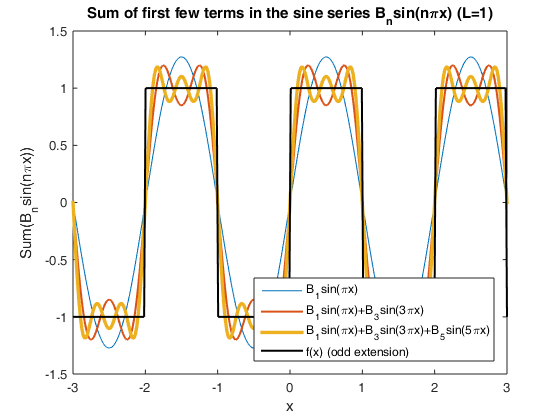# Fourier sine series: square wave

`Math 331, Fall 2017, Lecture 1, (c) Victor Matveev`

Fourier series of a constant function f(x)=1 converges to an odd periodic extension of this function, which is a square wave.

```clear;
hold off
L     = 1;                         % Length of the interval
x     = linspace(-3*L, 3*L, 300);  % Create 300 points on the interval [-3L, 3L]
Const = 4*L/pi;                    % Constant factor in the expression for B_n
Sn    = 0;                         % Initialize vector sum series to zero

for n = 1 : 2 : 5                  % Sum over odd terms only
Bn = Const/n;                  % Coefficients inversely proportional to n
Fn = Bn * sin(n*pi*x);         % Calculate Fourier term
Sn = Sn + Fn;                  % Add the term to Fourier sum
plot(x, Sn, 'linewidth', n/2); % Plot the fourier sum
hold on;
end

xlabel('x'); ylabel('Sum(B_nsin(n\pix))');
title('Sum of first few terms in the sine series B_nsin(n\pix) (L=1)');
plot(x, -1 + 2*(x>-2).*(x<-1) + 2*(x>0).*(x<1) + 2*(x>2).*(x<3),  'k-', 'linewidth', 1.5) % Tricky part of the code: generate square wave
legend('B_1sin(\pix)', 'B_1sin(\pix)+B_3sin(3\pix)', 'B_1sin(\pix)+B_3sin(3\pix)+B_5sin(5\pix)', 'f(x) (odd extension)', 'Location', 'Southeast');
```